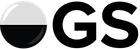# Proposal For Expected Score of Games that Exist Across Multiple Ratings Periods

This proposal has largely to do with correspondence ratings and rating updates.

There’s a weird assumption being made implicitly in our updates of correspondence games: the entire game might as well have taken place entirely in the most recent ratings period, as opposed to the months it took to actually complete, and that the rating and deviation described by the most recent ratings period is indicative of the entire span of time the game took place in. This effectively punishes players who improve while they have a corr game running.

Now, according to Anoek’s analysis of the data, correspondence strength is more useful for live/combined data than not using it, which is why we don’t need to scrap it entirely, but this weird assumption still bothers me.

So what do we do about it? Well, if we assume that the game is played at a steady pace across ratings periods (which makes most sense when these periods are time-discretized), and that the average move in a game has similar effect on result no matter which part of the game its in (this is a simplifying assumption, although likely does not make sense, given the effect of the opening far outweighs the effect of yose), we can then modify the E function to be based on the average (mean) rating of all ratings periods the game is in.

And that’s fine and dandy, but what RDs do we use in that situation? Well, we’re first going to do the smoothing process described in section 3.5 here so we don’t have an overestimate of the RD at the beginning of the game, then we’re going to try to find an estimate of the deviation of the mean rating.

So how do we estimate the deviation of this mean rating? Well, the first instinct would be to sum up the variances (RD2), take the average to find the standard deviation, then divide by sqrt(N) to find the standard error, but that assumes that all of these ratings are independently distributed, which they are not. Instead we’ll do something a little bit fancier. Let’s say if we knew the initial starting point perfectly (RD=0), then the average rating should, across the game, deviate by the standard error of volatilities across this time span. The standard error of this result should look like StE = sqrt(Σi=1Nσi2/N2), which, when incorporating the RD at the first ratings period should then calculate sqrt(StE2+RD2), where σi is the volatility at ratings period i, with 1 being the first ratings period in which the game takes place, and N being the last. which if you put into one calculation looks like sqrt(RD2+Σi=1Nσi2/N2).

Now, this whole process should be utilized solely for the sake of the E (Expectation), v, and delta functions (i.e.: only for calculations that involve predicting the outcome). They will affect rating, RD, and volatility, and there should likely be an investigation into exactly how it changes this, but for the sake of ease of calculation, I’m going for the relatively low-hanging fruit of the prediction itself.

There is one very questionable assumption I have not addressed yet, and that is the assumption that the RD at the start of the game is a better indicator than any other, or even averaging the deviations, or variances of all ratings periods. The reasoning is simplicity of understanding, although it should be just as reasonable to use any other RD (prior to adding the StE of volatilities) – and likely better to average the variances – I find it easier to understand from the standpoint of knowing the starting RD, given that you have gone through the smoothing process, and adding the StE as it is a process that moves forwards in time, although this is otherwise completely arbitrary.

I know that time-discretized ratings periods are being tested, and don’t imagine this should be incredibly hard to implement for testing (although I’m bad at coding so idk exactly) to see if it yields better results, so I figured this would be a good time to pitch the idea.

2 Likes